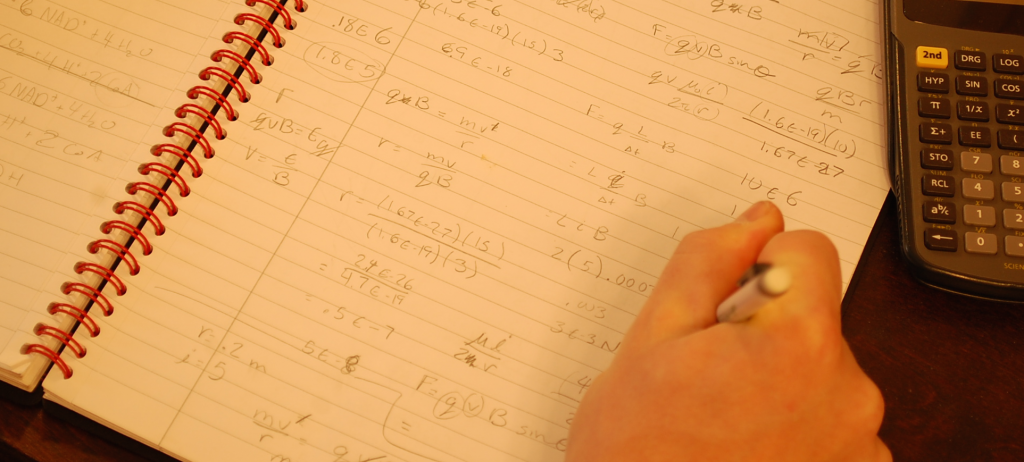Charlottesville
• (434) 296-5111
Great Falls
• (703) 759-3624
McLean
• (703) 288-2808# SAT Math

The SAT Math test covers a variety of arithmetic, algebra, and geometry concepts, with a particular focus on problem-solving skills. The emphasis on problem-solving in a fast-paced testing environment can pose challenges for strong and weak math students alike, so we wanted to outline this SAT section for test takers and parents.

## SAT Math Overview

The SAT’s Math test includes an assortment of subject matter from arithmetic, algebra, geometry, and basic probability. The SAT will always include at least three (possibly four, depending on the experimental section) sections of Math material. So what can students expect on these sections?

• An Emphasis on Problem Solving. The math concepts tested on the SAT aren’t particularly advanced. In fact, it generally includes material only through the middle of Algebra II, meaning that most juniors and seniors have seen all of the concepts by the time they take the SAT. The real challenge lies in how the test uses these concepts within word problems, diagrams, and other formats that can be counterintuitive to many students. The SAT Math test rewards flexibility and problem solving skills.
• Free Response Questions. Not all math questions on the SAT are multiple-choice. One section will include around 10 grid-in free response questions, to which students must supply their own answers. These questions aren’t designed to be more or less difficult than the rest of the SAT Math questions, but some students find them disorienting. Students should know that these questions are scored differently; there is no penalty for incorrect responses to free response math questions on the SAT.
• Progressively Harder Questions. In general, each section gets more difficult from start to finish, so students should budget their time accordingly to make sure they complete as many questions as possible. This is more of a trend than a rule of thumb, however; many sections will include a few easy questions near the end, and students often miss these because they spend too much time on earlier problems.
• Fast Pacing. On average, students get a little more than a minute per SAT Math question, which means they have to move quickly to score points. Simply skipping questions that would take too long can be an effective strategy that helps students maximize their scores. Students who approach complicated problems proactively (by marking up diagrams or redrawing figures, for example) also see good results on the SAT Math test.

## Math Content

The SAT Math test includes concepts from four major mathematical areas: arithmetic, algebra, geometry, and probability. A sampling of SAT math concepts included in each of these categories is listed below:

### Arithmetic

• Number Operations
• Number Properties
• Fractions, Decimals, and Percents
• Ratios and Rates
• Exponents and Roots
• Sequences and Series
• Direct and Inverse Variation
• Combinations and Permutations

### Algebra

• Word Problems
• Consecutive Integers
• Monomials, Binomials, and Polynomials
• Systems of Equations
• Functions
• Domain and Range
• Graphing
• Linear Equations

### Geometry

• Lines and Angles
• Triangles
• Other Polygons
• Circles
• Coordinate Geometry
• Solids
• Area and Volume

### Probability

• Basic Probability
• Mean, Median, Mode, and Range
• Sets
• Logic

## GLC’s Approach to SAT Math

The SAT Math Test can be challenging because it combines a wide variety of concepts with problem-solving skills in a condensed timeframe. In our prep, we prioritize fundamental concepts in a logical progression and teach a problem-solving approach that can boost results on this difficult test.

### Building Strong Foundations

The SAT doesn’t test math content that’s especially complicated or foreign, but it does test a lot of different concepts. It can be difficult for juniors and seniors to remember details from geometry classes they took several years before, so our SAT curriculum provides a thorough primer on the skills that slow students down most when they’re rusty. This is a particularly effective way to prepare for SAT Math, since many of the most complicated problems are actually just several simple concepts combined with one another.

### Strategic Time Management

Complex math problems on the SAT are dangerous because they’re usually so solvable. Students might get halfway through a problem before getting stuck and realizing that it will take five minutes to solve—time they can’t afford when the clock is ticking. We teach students how to identify complicated problems and work around them so they maximize the number of “easy points” they earn on each section, an approach that gets strong results while reducing stress.

### Developing Problem Solvers

Once students know the math concepts tested on the SAT, attention should turn to problem solving skills because the SAT loves to ask simple questions in confusing ways. We use real SAT example problems to demonstrate how to dissect complicated graphs, break diagrams into manageable chunks, and use questions against themselves in the search for solutions.

SAT tutoring helps students learn the fundamental concepts, problem solving skills, and confidence needed for top math scores. Click the button below to learn more about our program, view class schedules, or register for SAT Classes.